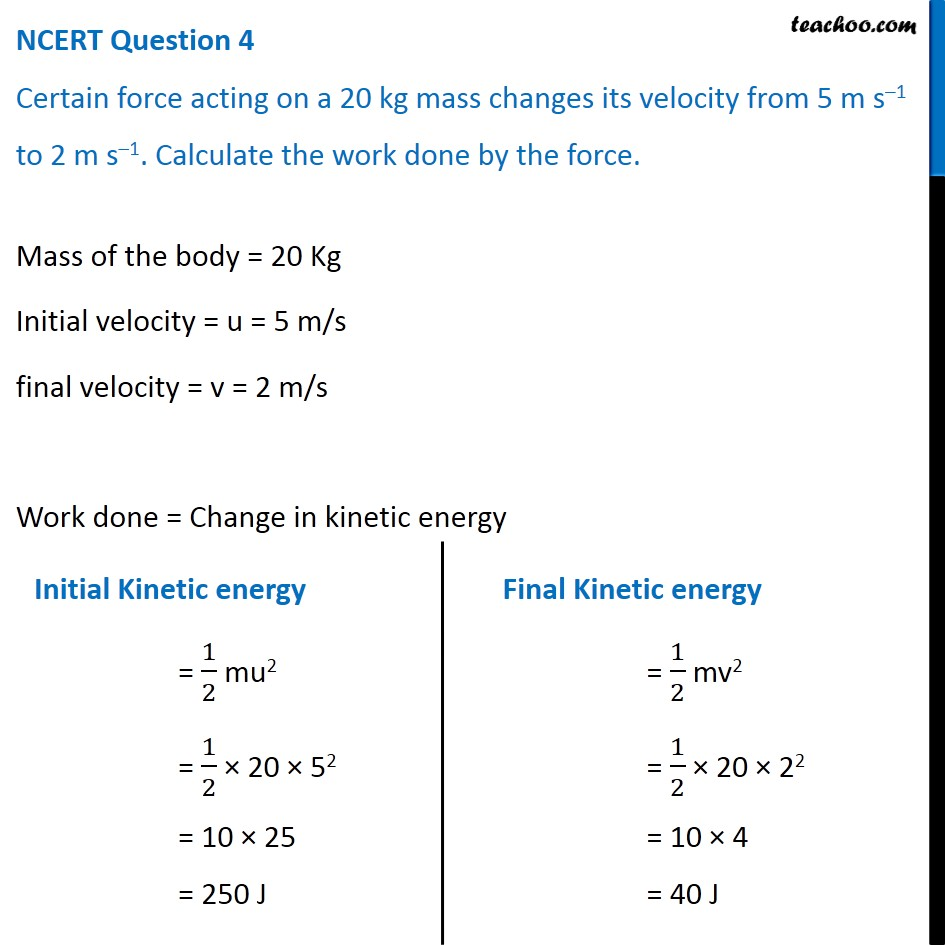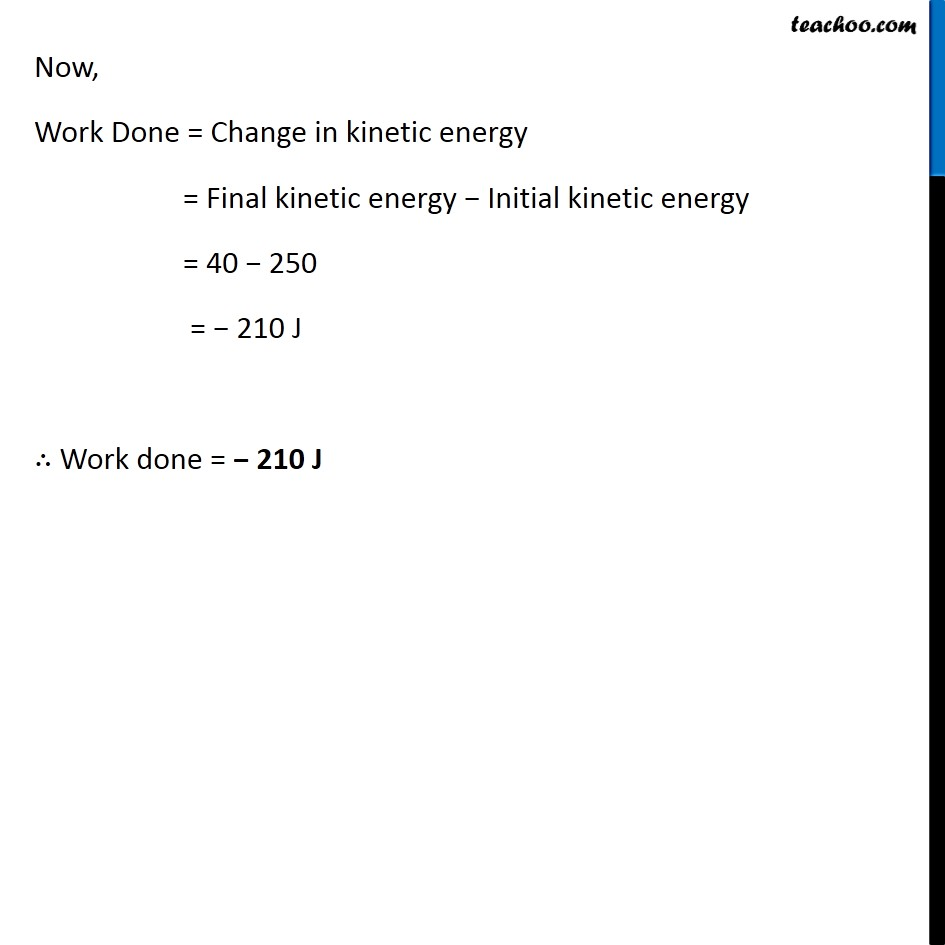NCERT Questions

Class 9
Chapter 11 Class 9 - Work and EnergyLearn in your speed, with individual attention - Teachoo Maths 1-on-1 Class

### Transcript

NCERT Question 4 Certain force acting on a 20 kg mass changes its velocity from 5 m s–1 to 2 m s–1. Calculate the work done by the force. Mass of the body = 20 Kg Initial velocity = u = 5 m/s final velocity = v = 2 m/s Work done = Change in kinetic energy Initial Kinetic energy = 1/2 mu2 = 1/2 × 20 × 52 = 10 × 25 = 250 J Final Kinetic energy = 1/2 mv2 = 1/2 × 20 × 22 = 10 × 4 = 40 J Now, Work Done = Change in kinetic energy = Final kinetic energy − Initial kinetic energy = 40 − 250 = − 210 J ∴ Work done = − 210 J